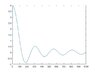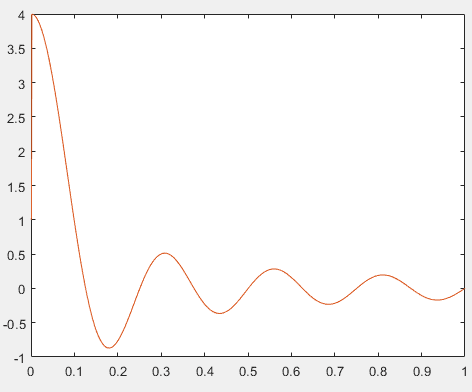# Plot with linspace: display x-tick values not index

skynelson
I want my graph to display the tick values along the x-axis, instead of the index number.
Because I use linspace, my data goes from 0 to 1, but my index ranges from 1 to 1000.
My plot labels the x-axis from 1 to 1000, by default. I want to change the default (or just program it) to display 0 to 1, so it looks like a physics graph. Any help much appreciated, thanks!
Code:
T = 4;
W = linspace(0, 1, 1000);
plot(sin(T*2*pi*W+1E-8)./(2*pi*W + 1E-8));Gold Member
This looks like Matlab to me - is that correct? If so, you just include the vector W insdied the plot command

plot(W, sin(T*2*pi*W+1E-8)./(2*pi*W + 1E-8));

jason

hdp12
Code:
A = plot(sin(T*2*pi*W+1E-8)./(2*pi*W + 1E-8));

A.Parent.XTick = [0:100:1000];
A.Parent.XTickLabel = {'0', '0.1', '0.2', '0.3', '0.4', '0.5', '0.6', '0.7', '0.8', '0.9', '1'};•skynelson
skynelson
This looks like Matlab to me - is that correct? If so, you just include the vector W insdied the plot command

plot(W, sin(T*2*pi*W+1E-8)./(2*pi*W + 1E-8));

jason
Yes, That's it! And yes, MATLAB. I figured there was a straightforward syntactical approach. Thanks Jason.

skynelson
Thanks hdp12 for the response! This is a good approach for more specific control over layout.

•hdp12